# Python datatime库详解（一码一图）

+关注继续查看

import datetime
import time
a=datetime.datetime.now()#打印现在的时间，详细的时间
print(a)
time.sleep(6)#延时6秒
b=datetime.datetime.now()#打印现在的时间
print(b)
print(b-a)#求出时间差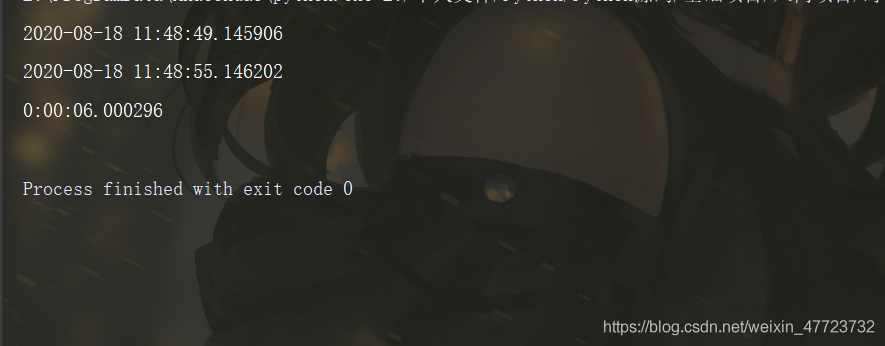c=datetime.datetime.now().date()#获取当前的日期，年月份
print(c)
d=datetime.datetime.now().timetuple()#获取每个时间的序列
print(d)
e=datetime.datetime.now().timetuple().tm_mon#获取月份（tm_year, tm_mon, tm_mday, tm_hour, tm_min, tm_sec, tm_wday, tm_yday, tm_isdst）
print(e)#还可以获取不同的值
f=datetime.datetime.now().date()+datetime.timedelta(days=2)
# 在我们现在的日期里面加入2天，最后打印出来，这里要注意，模块的使用，有优先级的混淆
print(f)
g=datetime.datetime.now()+datetime.timedelta(hours=2)
# 在现在的时间里面向后推迟2个小时
print(g)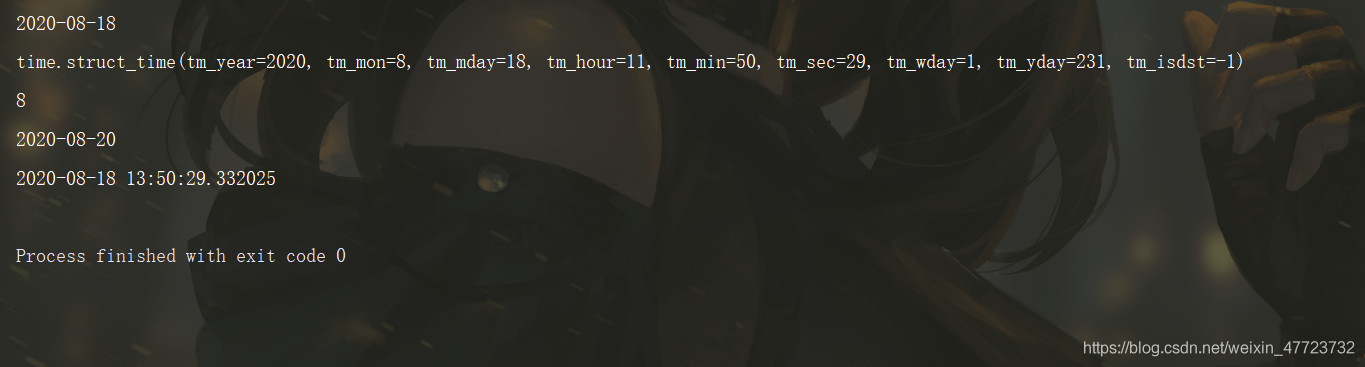f=datetime.date(day=1,month=datetime.date.today().month,year=datetime.date.today().year) -\
datetime.timedelta(days=1)
# 求出上一个月的最后一天日期，就用这个月的第一天减1天，这个里面的参数可以改动，默认为月份初始化天
print(f)
g=datetime.datetime.utcnow()#获取世界时间
print(g)
h=datetime.datetime.now()
print(h)
i=h-g#时差计算
print(i)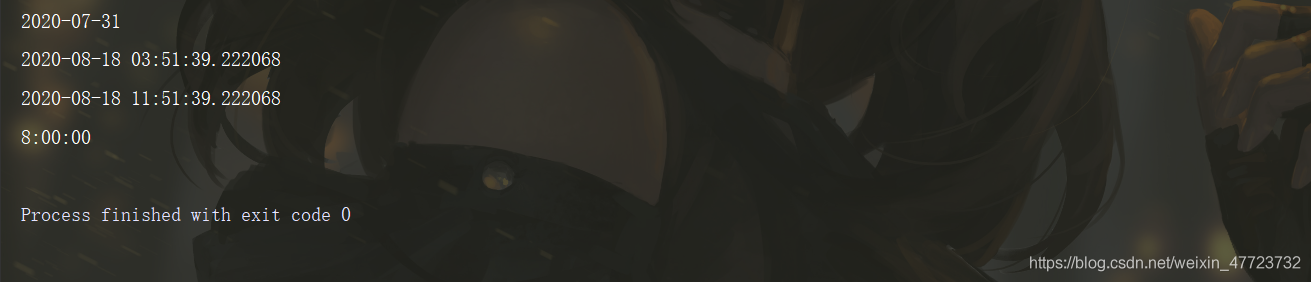j=datetime.datetime.now().strftime("%Y-%m-%d %H:%M:%S")#时间类型转为字符串类型
k=datetime.datetime.now()#时间类型
print(type(j))#显示类型
print(type(k))
print(j,end=";")
print(k)
# 看起来差不多，其实有很多差别，最基本的类型就不一样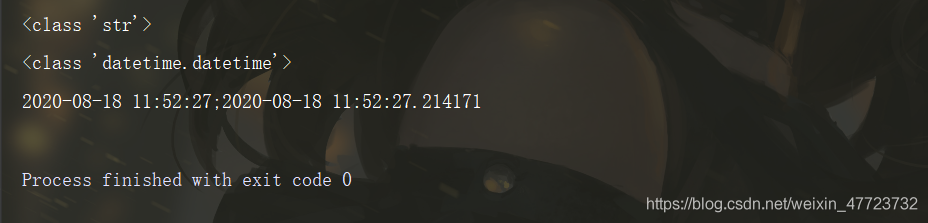l=datetime.datetime.strptime("2020-08-18 16:58:46","%Y-%m-%d %H:%M:%S")
# 字符串转为时间类型
print(l)
m=datetime.datetime.now().isocalendar()
# 返回这个日期是多少年，第几周，第几天
print(m)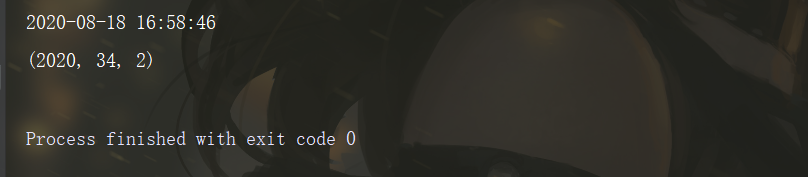input_date_str=input('请输入一个日期: ')
# 把输入的时间转换为时间类型
input_date=datetime.datetime.strptime(input_date_str,'%Y-%m-%d')
# 利用date.strftime("%j")  计算这个是第几天
days=int(input_date.strftime("%j"))#转换int类型
print(input_date_str+'是一年中的第 '+str(days)+' 天')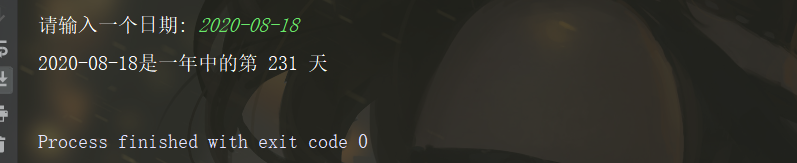# 显示为时间格式
print(datetime.time(21,28,14,123456))   # 21:28:14.123456
# 显示为日期格式
print(datetime.date(2019,12,14))    # 2019-12-14
# 显示为日期时间格式
print(datetime.datetime(2019,12,14,21,32,48,456))   # 2019-12-14 21:32:48.000456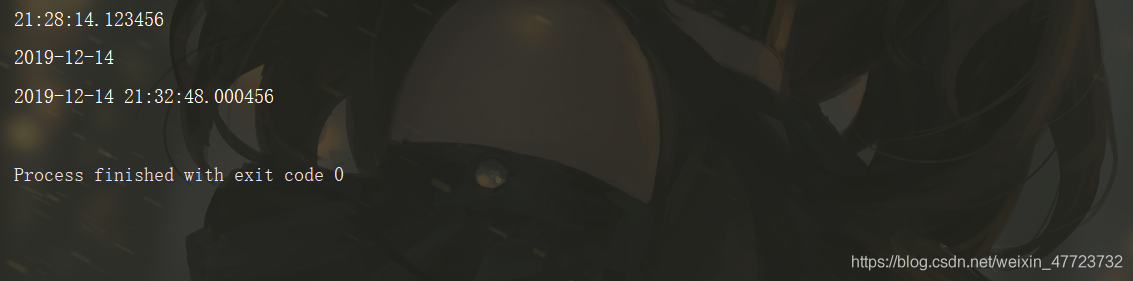# 通过日期算用户活了多久
user_birthday = [2001, 7, 1]
# 现在的时间 - 用户的生日
how_day = datetime.datetime.now() - datetime.datetime(*user_birthday)
print(how_day)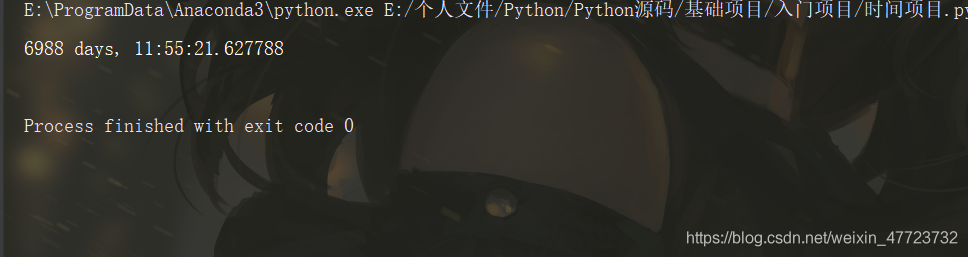print(datetime.datetime.now().timestamp())  # 时间戳的计算是从1900年1月1日0点开始的, 显示的数值单位为秒
print(datetime.datetime.fromtimestamp(1564229940.623349))   #时间戳转化为日期时间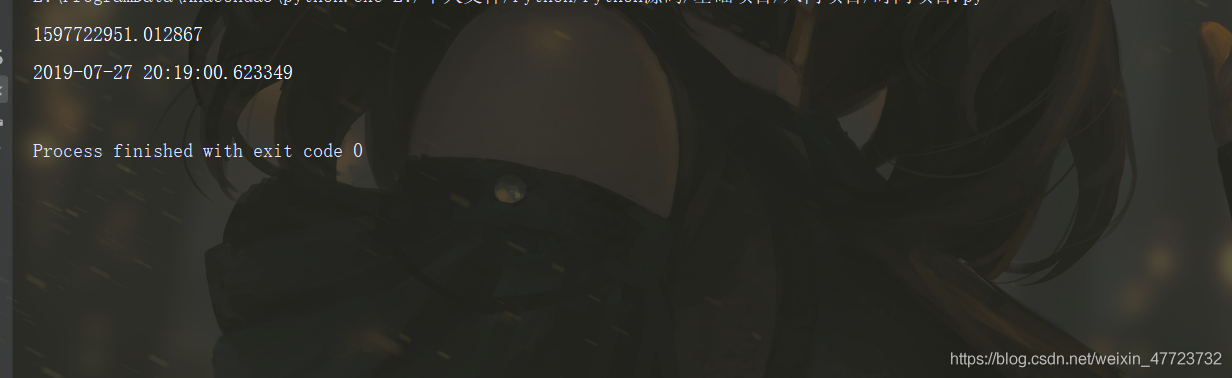now = datetime.datetime.now()
# 获取一天前的日期
print(now - datetime.timedelta(days=1))
# 获取1天20小时20分后的日期
print(now + datetime.timedelta(days=1, hours=20, minutes=20))
# timedelta的参数里面有days，hours，minutes......这些东西，我们可以自己定义然后进行相关运算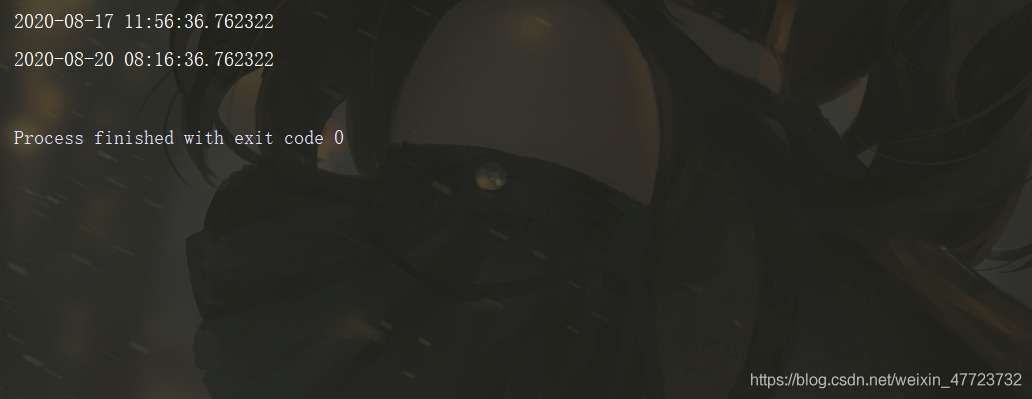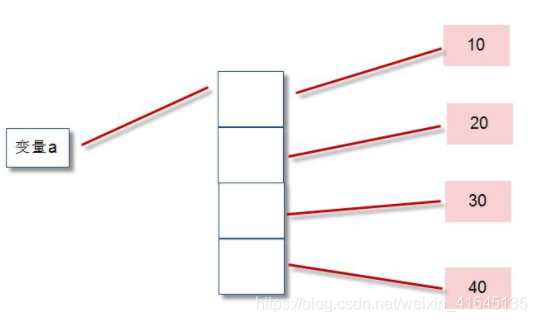Python列表详解
Python列表详解
28 0【愚公系列】2022年04月 Python教学课程 77-DRF框架之异常
【愚公系列】2022年04月 Python教学课程 77-DRF框架之异常
19 0Python3抓取糗百、不得姐

20488 0Java之调用Python代码
对于一个数据分析方面的工作者来说，最熟悉的语言无疑就是Python了。对于软件开发者而言，Java又是极其重要的工具。那么，一个很自然的问题就是，我们能够在Java中调用Python代码吗？   想要在Java中调用Python代码，有以下两个办法： 直接通过Runtime进行调用 调用Jython 在Java中如果需要调用第三方程序，可以直接通过Runtime实现，这也是最直接最粗暴的做法，粒度更加粗糙，效率较高，需要安装Python软件。
2390 0python 计时——time, datetime
2395 0723 0Python中单引号、双引号和三引号的区别
1271 0Python中单引号、双引号和三引号的区别
1118 0Python 入门教程 4 ---- Date and Time
第一节     1 介绍得到当前的时间datetime.now()     2 练习        1 设置变量now的值为datetime.
868 0

268

0